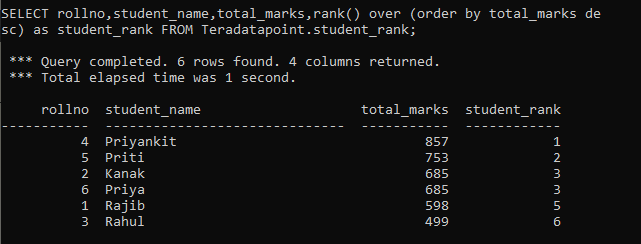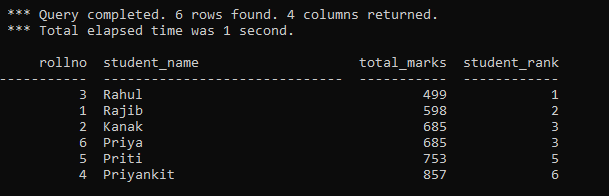Teradata RANK function assigns the ranking(1…n) of rows in the result set based on the value of the sort_expression list. The rows with the same value receive the same rank in Teradata.

You may also specify the sorting order in the RANK function. Descending order is the default order and assign the rank one to the largest value in the sorted list. Ascending order assigns rank one to the smallest value in the list.

The syntax of the Rank Function is as below.

`RANK ( sort_expression [ASC | DESC] );`

Where,

• sort_expression – This can be a literal or column expression or comma-separated list of literal or column expression.
• ASC – Ascending sort order.
• DESC – Descending sort order. The default sort order is DESC.

Consider the following student table of marks obtained by each student.

 rollno student_name total_marks 1 Rajib 598 2 Kanak 685 3 Rahul 499 4 Priyankit 857 5 Priti 753 6 Priya 685

We can now provide ranking to each student using the RANK function as below.

```SELECT rollno,student_name,total_marks,rank() over (order by total_marks de

*** Query completed. 6 rows found. 4 columns returned.
*** Total elapsed time was 1 second.

```

Output:-You can also assign the rank one to the student who secured the lowest marks as below.

```SELECT rollno,student_name,total_marks,rank(total_marks asc) as student_ran

*** Query completed. 6 rows found. 4 columns returned.
*** Total elapsed time was 1 second.```

Output:-### Table Preparation of the above Example

```ct teradatapoint.student_rank
(
rollno int,
student_name varchar(30),
total_marks int
)primary index(rollno);